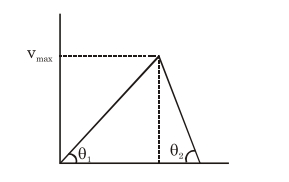# A scooter accelerates from rest for time`
Question:

A scooter accelerates from rest for time $t_{1}$ at constant rate $\mathrm{a}_{1}$ and then retards at constant rate $a_{2}$ for time $t_{2}$ and comes to rest. The correct

value of $\frac{t_{1}}{t_{2}}$ will be :-

1. $\frac{a_{1}+a_{2}}{a_{2}}$

2. $\frac{\mathrm{a}_{2}}{\mathrm{a}_{1}}$

3. $\frac{\mathrm{a}_{1}}{\mathrm{a}_{2}}$

4. $\frac{a_{1}+a_{2}}{a_{1}}$

Correct Option: , 2

Solution:

Draw vt curve$\tan \theta_{1}=a_{1}=\frac{v_{\max }}{t_{1}}$

$\& \tan \theta_{2}=\mathrm{a}_{2}=\frac{\mathrm{v}_{\max }}{\mathrm{t}_{2}}$

$\div$ above

$\frac{t_{1}}{t_{2}}=\frac{a_{2}}{a_{1}}$